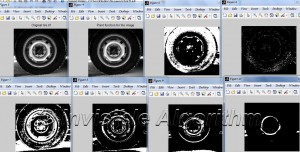# Matlab – Point Functions and Histogram Image SegmentationIn image processing point operation or function results in new histogram different from the original image. It is basically taking average of several points and make it into one points such as b(i,j) = 10 round (A(i,j) / 10). You can clearly see the difference in histogram view. Image segmentation somehow related to point functions. A scene in an image composed of several different regions, object and etc. Segmentation is decomposition of an image into these regions or objects. As a human, we can easily identify each of object in an image. However, automatic segmentation using computer algorithm is difficult and right now consider unresolved. Automatic segmentation of image is still actively researched.

Histogram based image segmentation is based on point processing. It’s just a simple algorithm to have initial guess to segment an image based on the brightness of each point in the image.

Below is the Matlab code for histogram based image segmentation.

a1=10*round(a/10);
figure,imhist(a);
figure,imhist(a1);

figure,imshow(a), title(‘Original tire.tif’);
figure, imshow(a1), title(‘Point function of the image’);

a2=255*((a>=0)&(a<=14));figure, imshow(a2);
a3=255*((a>=15)&(a<=15));figure, imshow(a3);
a4=255*((a>=16)&(a<=99));figure, imshow(a4);
a5=255*((a>=100)&(a<=149));figure, imshow(a5);
a6=255*((a>=150)&(a<=220));figure, imshow(a6);
a7=255*((a>=221)&(a<=255));figure, imshow(a7);

That matlab code will do. Try to execute it. The first and second figure is the histogram of the original image and the same image after point functions or point processing.The next figure will show the original image, the image after point functions and six different images using histogram based image segmentation.That’s all. Thank You.

Posted on Categories Matlab